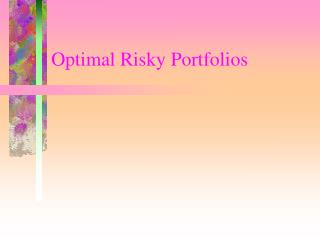# Optimal Risky Portfolios - PowerPoint PPT PresentationDownload PresentationOptimal Risky Portfolios

Optimal Risky PortfoliosDownload Presentation## Optimal Risky Portfolios

- - - - - - - - - - - - - - - - - - - - - - - - - - - E N D - - - - - - - - - - - - - - - - - - - - - - - - - - -
##### Presentation Transcript

1. Optimal Risky Portfolios

2. Objective • Objective - finding the optimal risky portfolio • Start with two risky assets • find portfolios with lowest possible risk, given a set of assets • find the tangency portfolio based on the set of risky assets and the risk-free asset • Generalize to more than two risky assets

3. Weighted sums of random variables: Applied in portfolio theory • E(rp)=w1E(r1)+w2E(r2), • VAR(rp)= w1212+ w2222+2 w1w212= w1212+ w2222+2 w1w2121

4. Example: Assuming the correlation between East Co. and West Co. is 0.6, and portfolio weights for East Co. and West Co. are 0.25 and 0.75 respectively.. E(rp)=0.25E(rE)+0.75E(rW)=0.25*11.44%+0.75*9.52%=0.10 VAR(rp)= 0.252E2+ 0.752W2+2 0.25*0.75EWEW =0.252*9.0181%+ 0.752*0.7851%+2 0.25*0.75*0.6*30.0301%*8.8606% =7.1158% rp=10.585%

5. Minimum-Variance Weights for Two Asset Portfolio

6. Example - Problem Statement Given the following information, what is the expected return and standard deviation for the minimum-variance portfolio?

7. Example - Minimum-Variance Portfolio Weights

8. Example - Portfolio Return and Standard Deviation • E(R) = .8873(.06) + .1127(.15) = .0701 • Var = (.8873)2(.12)2 + (.1127)2(.21)2 + 2(.8873)(.1127)(.01008)Var = .01391 • Std. Dev. = .11795

9. Investment Set • Efficient Frontier • The portfolio that provides the highest return for a given level of risk • For a risky portfolio this is the upper portion of the curve beginning at the minimum-variance portfolio • For a combination of a risk-free asset and a risky portfolio it is the tangency line emanating from the risk-free return

10. Findng the Tangency Portfolio From previous example, assume risk-free rate is 4%.

11. Another Example - Problem Statement Suppose the risk-free raet of return is 5% and you have two risky assets, Cynthia Enterprises and Tasha’s Toys. They have the following expected returns: E(RCE) = .15 and E(RTT) = .25. Covariance Matrix

12. Example - Minimum-Variance Risk and Return • E(R) = .8048(.15) + .1952(.25) = .1695 • Var = (.8048)2(.0169) + (.1952)2(.0441) + 2(.8048)(.1952)(.00819) = .0152 • Std. Dev. = .1233 • Reward-to-variability = (.1695 - .05)/.1233 = .9694

13. Capital Allocation Decision • Suppose you have \$10,000 to invest and you want to earn an expected return of 17%. How much should you invest in each asset? • .17 = .1980y + (1-y)(.05)y = .6060 • Investment • (1-.6060)(10,000) = 3940 in T-bills • (.6060)(.4802)(10,000) = 2910 in Tasha’s Toys • (.6060)(.5198)(10,000) = 3150 in Cynthia’s

14. Markowitz Portfolio Selection Model • Generalize the two asset case to many risky assets and a risk-free security • Identify the risk-return combinations • Identify the optimal risky portfolio - steepest CAL • Choose the complete portfolio based on preferences

15. Introduce Risk-free Asset • Vary CAL until the tangency portfolio is found • This is the optimal portfolio for ALL investors regardless of risk preferences for this given set of assets. • Investor imposed constraints prevent everyone from holding the same risky portfolio • The more risk averse the investor, the more they will hold of the risk-free asset

16. Separation Property • Investment decision is separated into two parts • technical determination of optimal risky portfolio • decision on investment between risky portfolio and risk-free asset based on personal preference • This property makes professional money management very efficient.